Question

# a) The rotor of a helicopter is gaining angular speed with constant angular acceleration. At t...

a) The rotor of a helicopter is gaining angular speed with constant angular acceleration. At t = 0 it is rotating at 1.25 rad/s. From t = 0 to t = 2.00 s, the rotor rotates through 8.00 rad. What is the angular acceleration of the rotor?

b) Through what angle (in radians) does the rotor rotate from t = 0 to t = 4.00 s? in radians

c) Shortly after a vinyl record (radius 0.152 m) starts rotating on a turntable, its angular velocity is 1.60 rad/s and increasing at a rate of 8.00 rad/s2. At this instant, for a point at the rim of the record, what is the tangential component of acceleration?

d) At this instant, for a point at the rim of the record, what is the centripetal component of acceleration?

e) At this instant, for a point at the rim of the record, what is the magnitude of acceleration?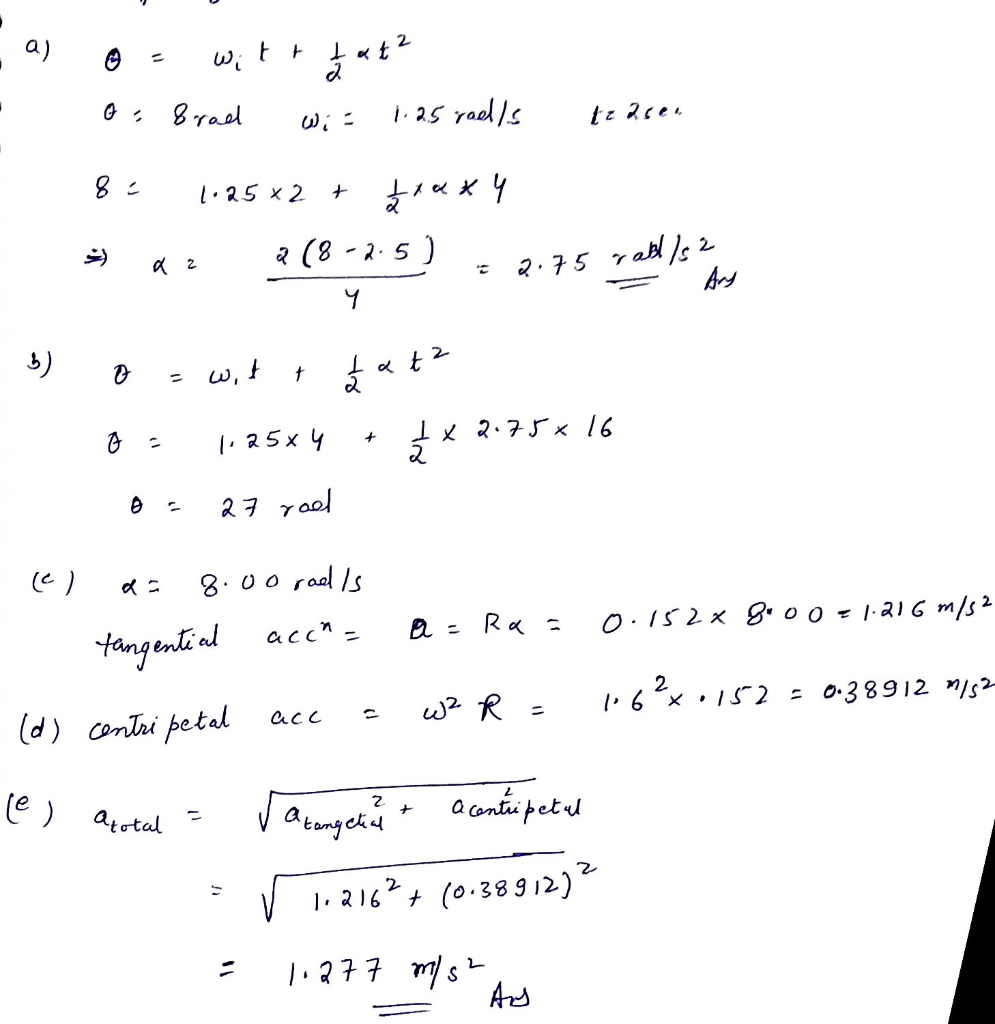#### Earn Coins

Coins can be redeemed for fabulous gifts.

Similar Homework Help Questions
• ### A wheel 1 m in radius starts rotating from rest with a constant angular acceleration of...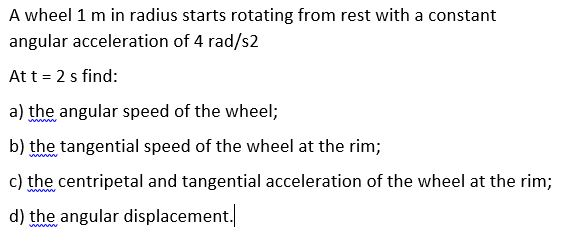A wheel 1 m in radius starts rotating from rest with a constant angular acceleration of 4 rad/s2 At t = 2 s find: a) the angular speed of the wheel; b) the tangential speed of the wheel at the rim; c) the centripetal and tangential acceleration of the wheel at the rim; d) the angular displacement.

• ### A disk rotates with constant angular acceleration. The initial angular speed of the disk is 2.1π...

A disk rotates with constant angular acceleration. The initial angular speed of the disk is 2.1π rad/s. After the disk rotates through 13.6π radians, the angular speed is 6.2π rad/s. (a) What is the magnitude of the angular acceleration? (b) How much time did it take for the disk to rotate through 13.6π radians? (c) What is the tangential acceleration of a point located at a distance of 9.9 cm from the center of the disk?

• ### Problem 2:3 pa velocity vis and a cons 2:(5 pts) xith a diameter of 0.75 m is rotating about a fix axis with an initial angular velocity of 0.25 rev/s and a constant angular acceleration of 0.9 r...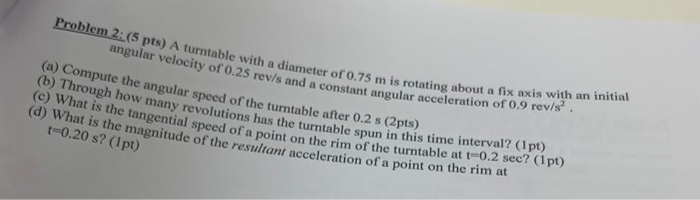Problem 2:3 pa velocity vis and a cons 2:(5 pts) xith a diameter of 0.75 m is rotating about a fix axis with an initial angular velocity of 0.25 rev/s and a constant angular acceleration of 0.9 revis. (a) Compute the angular speed of the turntable after 0.2 s (2pts (o) Through how manar spced of the turntable after 0.2 s (Opts) many revol utions has the turntable spun in this time interval? (1pt) al speed of a point on...

• ### A helicopter blade has an angular speed of 40.8 rad/s and an angular acceleration of 8.17...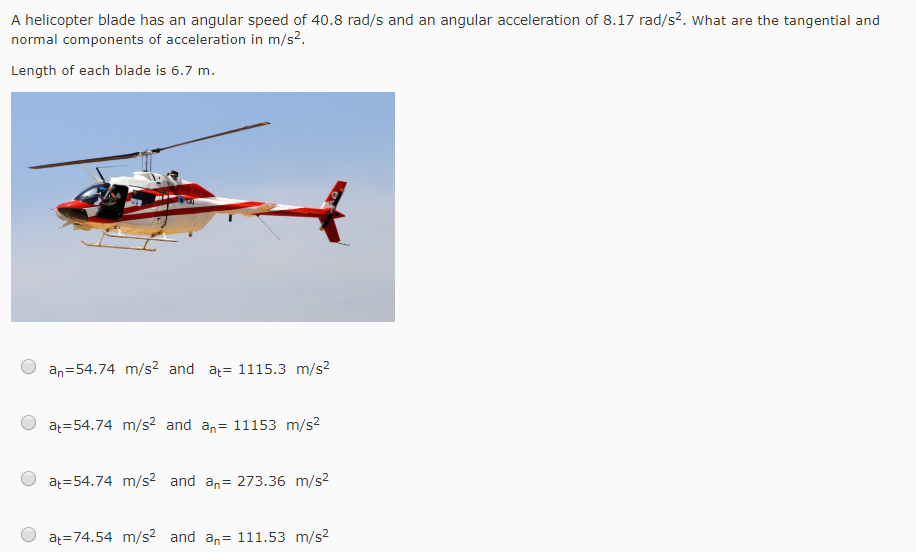A helicopter blade has an angular speed of 40.8 rad/s and an angular acceleration of 8.17 rad/s2. What are the tangential and normal components of acceleration in m/s Length of each blade is 6.7 m. O an-54.74 m/s2 and a 1115.3 m/s2 O a 54.74 m/s2 and an- 11153 m/s2 O at 54.74 m/s2 and an 273.36 m/s2 O at 74.54 m/s2 and an 111.53 m/s2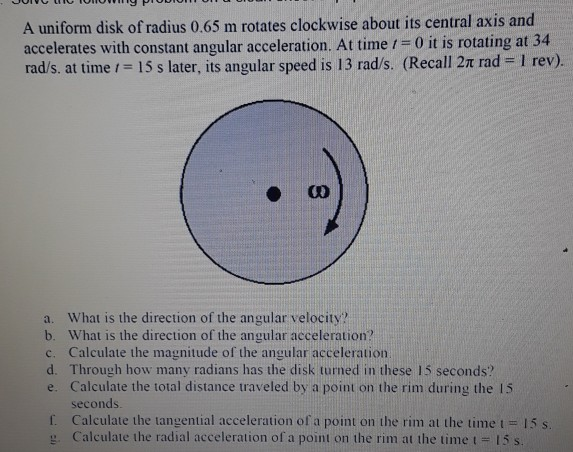please help A uniform disk of radius 0.65 m rotates clockwise about its central axis and accelerates with constant angular acceleration. At time 1-0 it is rotating at 34 rad/s, at timeに15 s later, its angular speed is 13 rad/s. (Recall 2π rad-1 rev). a. What is the direction of the angular velocity? b. What is the direction of the angular acceleration? c. Calculate the magnitude of the angular acceleration d. Through how many radians has the disk turned in...

• ### A 36.2-cm diameter disk rotates with a constant angular acceleration of 2.8 rad/s2. It starts from...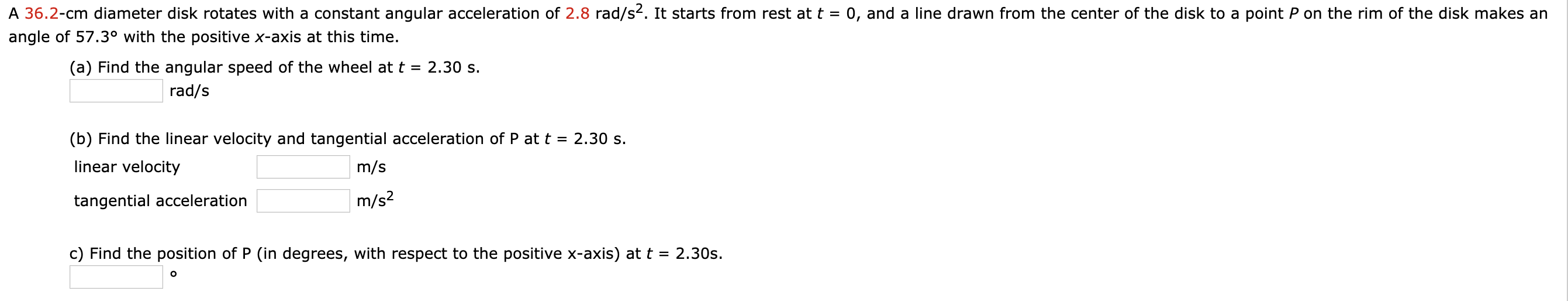A 36.2-cm diameter disk rotates with a constant angular acceleration of 2.8 rad/s2. It starts from rest at t = 0, and a line drawn from the center of the disk to a point P on the rim of the disk makes an angle of 57.3° with the positive x-axis at this time. (a) Find the angular speed of the wheel at t = 2.30 s. rad/s (b) Find the linear velocity and tangential acceleration of P at t =...

• ### A 39.2-cm diameter disk rotates with a constant angular acceleration of 2.8 rad/s2. It starts from rest at t = 0, a...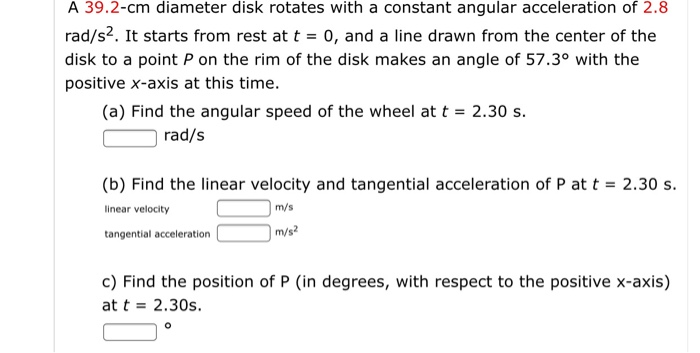A 39.2-cm diameter disk rotates with a constant angular acceleration of 2.8 rad/s2. It starts from rest at t = 0, and a line drawn from the center of the disk to a point P on the rim of the disk makes an angle of 57.3° with the positive x-axis at this time. (a) Find the angular speed of the wheel at t 2.30 s. rad/s 2.30 s (b) Find the linear velocity and tangential acceleration of P at t...

• ### 16-25. The disk starts from rest and is given an angular acceleration a = (1001/3) rad/s?,...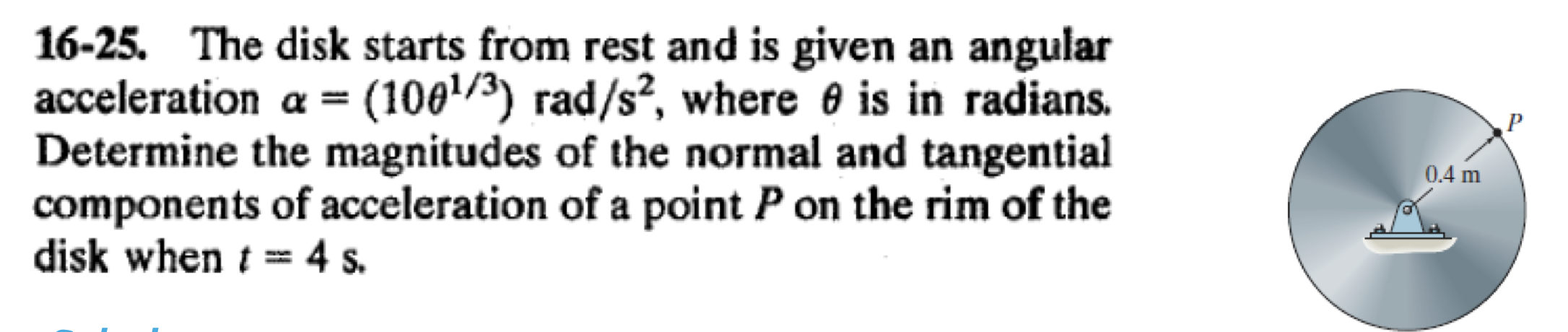16-25. The disk starts from rest and is given an angular acceleration a = (1001/3) rad/s?, where @ is in radians. Determine the magnitudes of the normal and tangential components of acceleration of a point P on the rim of the disk when t = 4 s. 0.4 m

• ### The blades of a helicopter rotor are 3.4m long from the central shaft to the tip...

The blades of a helicopter rotor are 3.4m long from the central shaft to the tip of each blade. During a performance test, the rotational speed of the rotor is 530 revolutions/minute. What is the tangential speed of the rotor? What is the centripetal acceleration of the blade tip expressed in g? 1 g is 9.8m/s^2

• ### A 47.4-cm diameter disk rotates with a constant angular acceleration of 2.80 rad/s2. It starts from...

A 47.4-cm diameter disk rotates with a constant angular acceleration of 2.80 rad/s2. It starts from rest at t = 0, and a line drawn from the center of the disk to a point P on the rim of the disk makes an angle of 57.3° with the positive x-axis at this time. (a) Find the angular speed of the wheel at t = 2.30 s. rad/s (b) Find the linear velocity and tangential acceleration of P at t =...